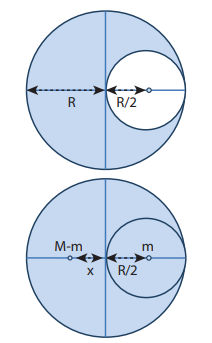Home | | Physics 11th std | Solved Example Problems for Center of Mass of Two Point Masses

# Solved Example Problems for Center of Mass of Two Point Masses

Physics : Motion of System of Particles and Rigid Bodies : Center of Mass of Two Point Masses

Solved Example Problems for Center of Mass of Two Point Masses

### Example 5.1

Two point masses 3 kg and 5 kg are at 4 m and 8 m from the origin on X-axis. Locate the position of center of mass of the two point masses (i) from the origin and (ii) from 3 kg mass.

### Solution

Let us take, m1 = 3 kg and m2= 5 kg

### (i) To find center of mass from the origin:

The point masses are at positions, x1 = 4 m, x2 = 8 m from the origin along X axis.The center of mass xCM can be obtained using equation 5.4.The center of mass is located 6.5 m from the origin on X-axis.

### (ii) To find the center of mass from 3 kg mass:

The origin is shifted to 3 kg mass along X-axis. The position of 3 kg point mass is zero (x1 = 0) and the position of 5 kg point mass is 4 m from the shifted origin (x2 = 4 m).The center of mass is located 2.5 m from 3 kg point mass, (and 1.5 m from the 5 kg point mass) on X-axis.

This result shows that the center of mass is located closer to larger mass.

If the origin is shifted to the center of mass, then the principle of moments holds good.

m1x1=m2x2; 3x2.5=5x1.5;7.5=7.5

When we compare case (i) with case (ii), the xCM = 2.5m from 3 kg mass could also be obtained by subtracting 4 m (the position of 3 kg mass) from 6.5 m, where the center of mass was located in case (i)

### Example 5.2

From a uniform disc of radius R, a small disc of radius R/2 is cut and removed as shown in the diagram. Find the center of mass of the remaining portion of the disc.

### Solution

Let us consider the mass of the uncut full disc be M. Its center of mass would be at the geometric center of the disc on which the origin coincides.

Let the mass of the small disc cut and removed be m and its center of mass is at a position R/2 to the right of the origin as shown in the figure.Hence, the remaining portion of the disc should have its center of mass to the left of the origin; say, at a distance x. We can write from the principle of moments,If σ is the surface mass density (i.e. mass per unit surface area), σ=M/πR2; then, the mass m of small disc is,The center of mass of the remaining portion is at a distance R/6 to the left from the center of the disc.

If, the small disc is removed concentrically from the large disc, what will be the position of the center of mass of the remaining portion of disc?

### Example 5.3Study Material, Lecturing Notes, Assignment, Reference, Wiki description explanation, brief detail
11th Physics : UNIT 5 : Motion of System of Particles and Rigid Bodies : Solved Example Problems for Center of Mass of Two Point Masses |